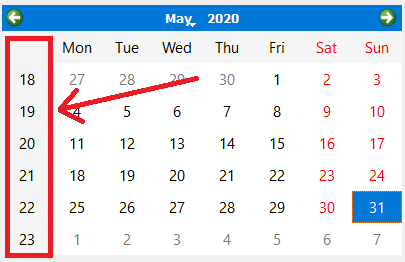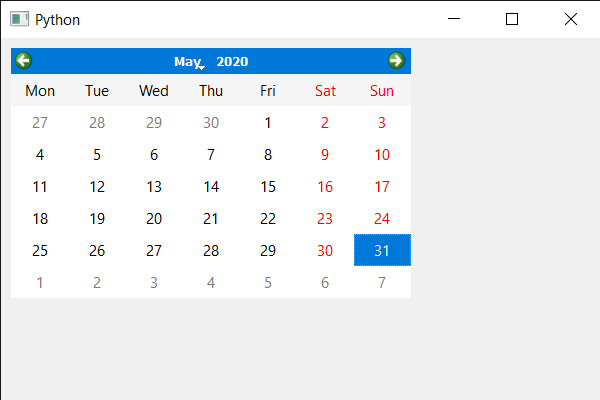Open In App

# PyQt5 QCalendarWidget – Setting Vertical header format

In this article we will see how we can set the vertical header format of the QCalendarWidget. Vertical header is the part of QCalendarWidget that tells the ISO week numbers, below is the representation of how vertical header looks like.There are basically two formats available for the vertical header one is the ISOWeekNumbers, its value is 1 and it is the default one second is the NoVerticalHeader its value is 0 and it do not display the vertical header.

In order to do this we will use setVerticalHeaderFormat method with the QCalendarWidget object.
Argument : It takes vertical header format as argument
Return : It returns None

Below is the implementation

## Python3

 `# importing libraries``from` `PyQt5.QtWidgets ``import` `*``from` `PyQt5 ``import` `QtCore, QtGui``from` `PyQt5.QtGui ``import` `*``from` `PyQt5.QtCore ``import` `*``import` `sys`  `class` `Window(QMainWindow):` `    ``def` `__init__(``self``):``        ``super``().__init__()` `        ``# setting title``        ``self``.setWindowTitle(``"Python "``)` `        ``# setting geometry``        ``self``.setGeometry(``100``, ``100``, ``600``, ``400``)` `        ``# calling method``        ``self``.UiComponents()` `        ``# showing all the widgets``        ``self``.show()` `    ``# method for components``    ``def` `UiComponents(``self``):` `        ``# creating a QCalendarWidget object``        ``calendar ``=` `QCalendarWidget(``self``)` `        ``# setting geometry to the calendar``        ``calendar.setGeometry(``10``, ``10``, ``400``, ``250``)` `        ``# setting vertical header format``        ``calendar.setVerticalHeaderFormat(QCalendarWidget.NoVerticalHeader)` `       `  `# create pyqt5 app``App ``=` `QApplication(sys.argv)` `# create the instance of our Window``window ``=` `Window()` `# start the app``sys.exit(App.``exec``())`

Output :